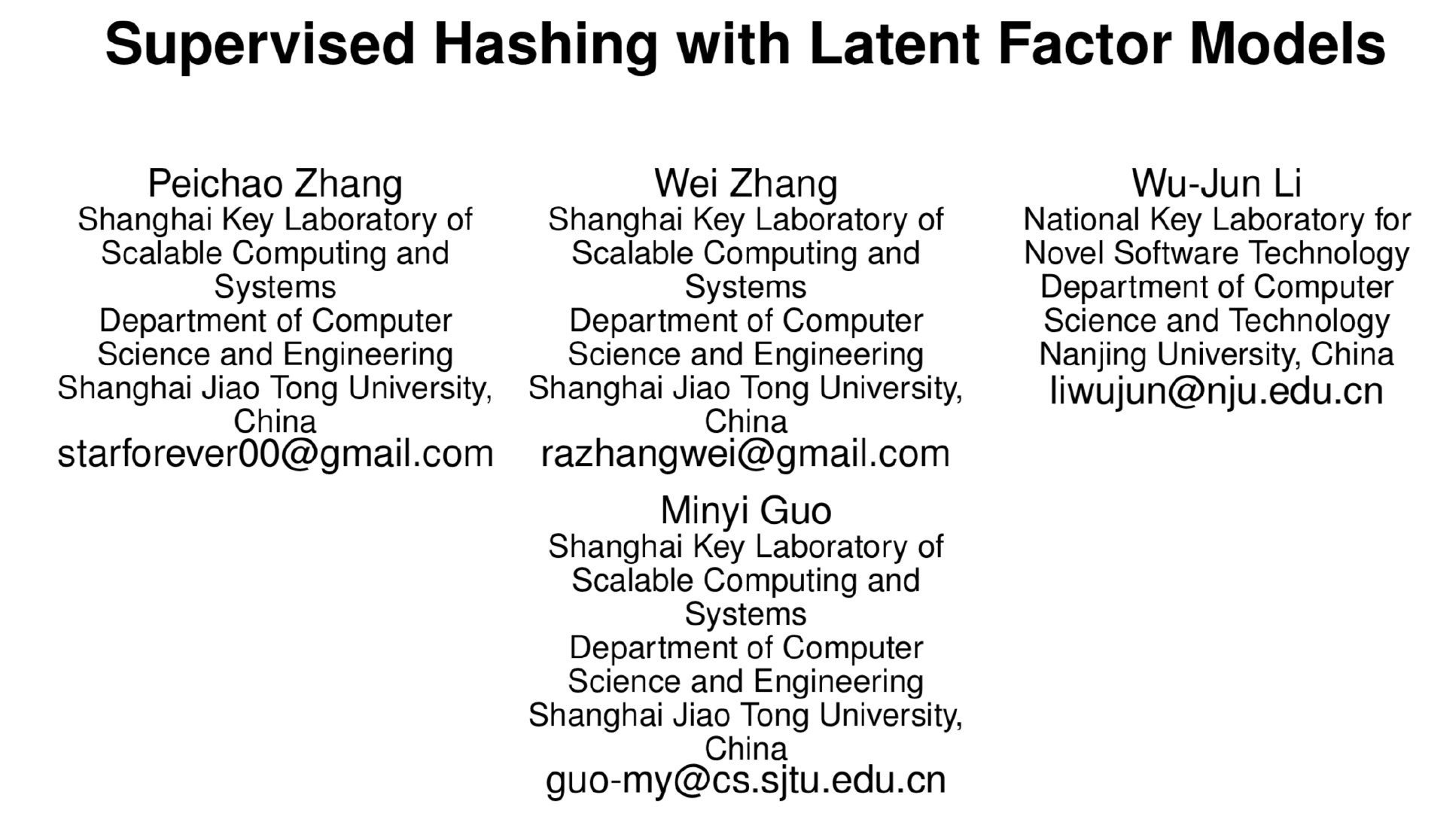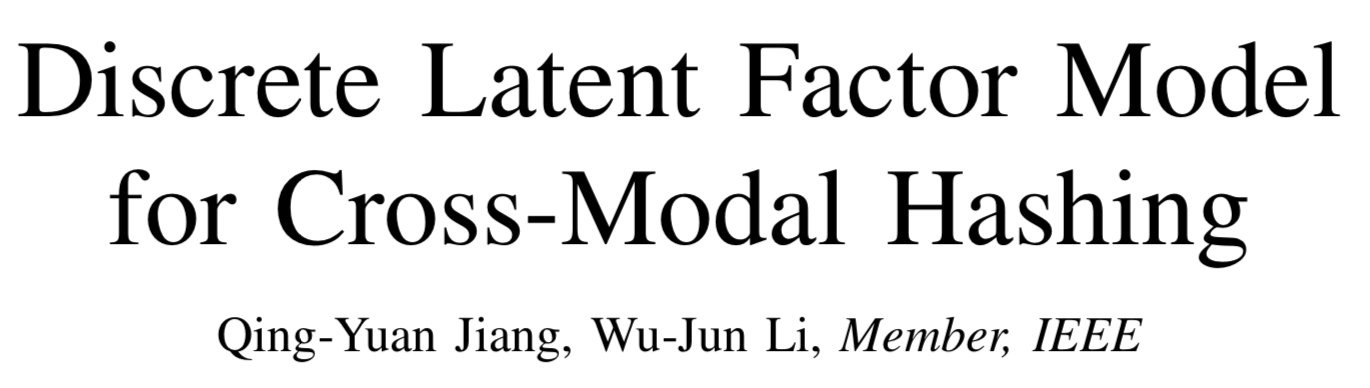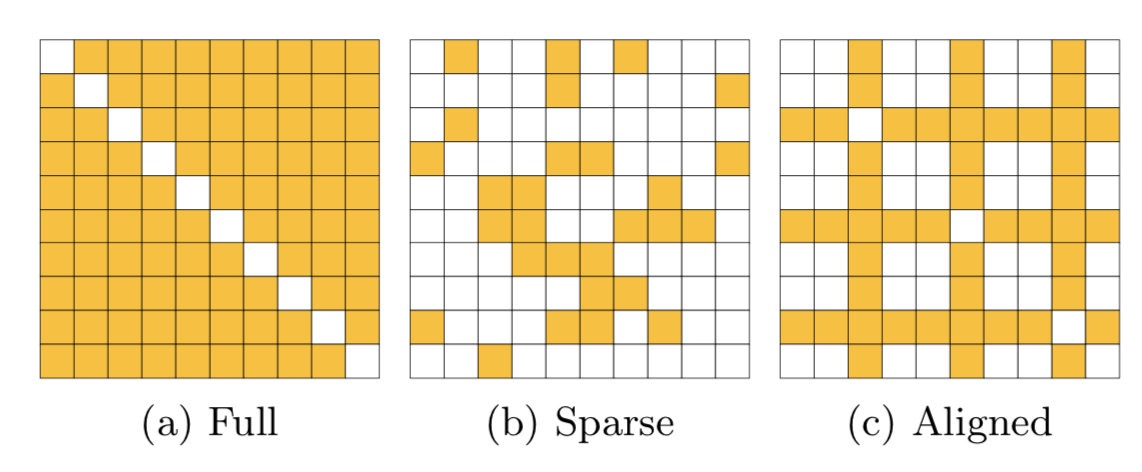### LFH

#### 优化

$\mathbf{U}_{i*}(t)$ 表示某个时间步 $t$$\mathbf{U}_{i*}$ 的值，为了使 $\widetilde{L}\left(\mathbf{U}_{i *}\right)$ 最大，只需要令 $\widetilde{L}\left(\mathbf{U}_{i *}\right)$$\mathbf{U}_{i*}^T$ 的梯度为0，即可得到：### DLFH

1. DLFH 进行的是跨模态哈希，而 LFH 则是单模态哈希
2. DLFH 是一种离散优化方法，直接学到二进制哈希码，而 LFH 则将二值放松到了实值
3. 第三点是更新方式的不同，LFH 一次更新一个数据点的哈希码，而 DLFH 一次更新所有数据点的某一位哈希码，具体的更新方式在下文中介绍

#### 方法

DLFH 的目标就是最大化对数似然，即优化下面的目标函数：

#### 优化

DLFH 每次优化 $\mathbf{U}$ 的一列，即 $\mathbf{U}_{*k}$，为了达到这个目的，需要建立 $L(\mathbf{U}_{*k})$ 的一个下界，然后最大化这个下界。首先计算目标函数 $L$ 对于 $\mathbf{U}_{*k}$ 的梯度以及 Hessian 矩阵如下：

$\mathbf{U}_{* k}(t)$ 表示第 $t$ 个迭代时 $\mathbf{U}_{* k}$ 的值，$\frac{\partial L}{\partial \mathbf{U}_{* k}}(t)$ 表示 $\mathbf{U}_{* k}(t)$ 的梯度，$\mathbf{H} = -\frac{n\lambda^2}{4c^2}\mathbf{I}$，定义 $\widetilde{L}\left(\mathbf{U}_{* k}\right)$ 如下：

### 参考文献

Zhang, Peichao, et al. “Supervised hashing with latent factor models.” Proceedings of the 37th international ACM SIGIR conference on Research & development in information retrieval. ACM, 2014.

Jiang, Qing-Yuan, and Wu-Jun Li. “Discrete Latent Factor Model for Cross-Modal Hashing.” IEEE Transactions on Image Processing (2019).

https://en.wikipedia.org/wiki/Matrix_calculus

https://en.wikipedia.org/wiki/Hessian_matrix

### 附录

$\widetilde{L}\left(\mathbf{U}_{i *}\right)$$\mathbf{U}_{i*}^T​$ 的梯度为0，即